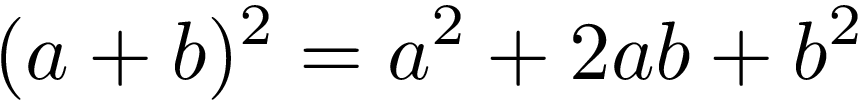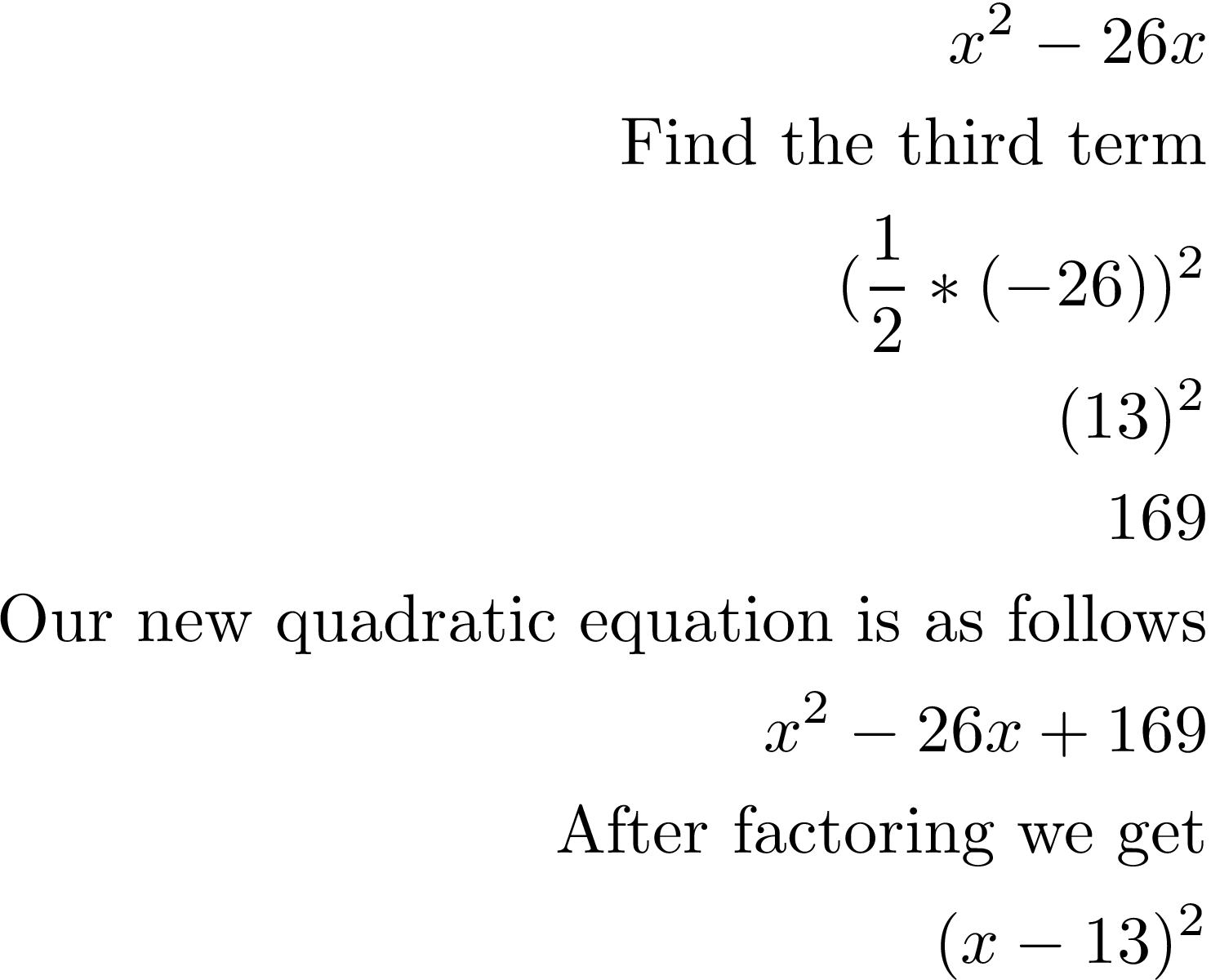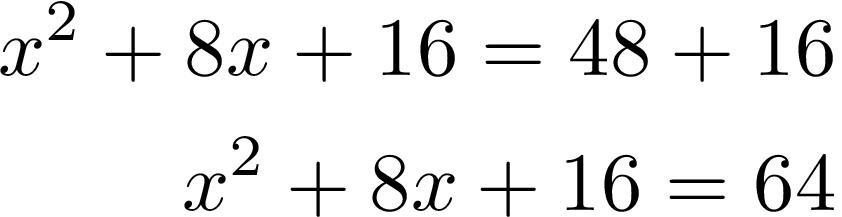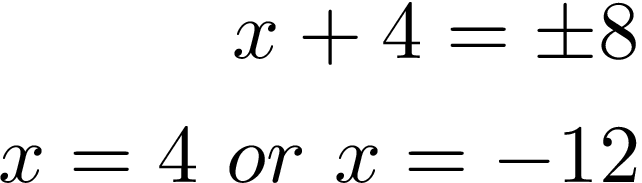# Completing the Square

One method for solving quadratic equations is called completing the square. This approach is a little confusing but we will try to work through it together in this post.

What is Completing a SquareCompleting the square is used when your quadratic equation is not a perfect square. Below is an example of a perfect square quartic formula =. The first is in the standard quadratic form and the second is after it has been simplified.However, not all equations are this easy, consider the example below.There is no quick way to factor this as there is no perfect square. We have to use something called the binomial square pattern.This is where it gets confusing but essential what the binomial square pattern is saying is that if you want to find the third term (b squared) you must take the second term and multiple it by1/2. We multiplied by 1/2 because this is the reciprocal of multiplying by 2 as shown in the equation. Lastly, we square this value. Below is the application of what we just learned from our problem equation.By taking the second term, multiplying by 1/2 and squaring it we were able to create the trinomial we needed to create the perfect square. By doing this we also solved for x if this was a full equation.

Examples

When using the completing the square approach with a quadratic formula there are some additional steps. We will work through an example belowWe are missing the third term and we need to find this first. Our second term is 8 so we will plug this in to find the third termWe take this number 16 and add it to both sides which is a rule whenever manipulating an equation. Therefore, we get the following.We can now factor the left side as shown below.To remove the square we need to square root both sides. In other words, we are employing the use of the square root property.